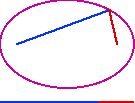## nafeducation.org

This term has distinctly different definitions for different authors.

Usage 1: For some authors, this refers to the distance from the center to the focus for either an ellipse or a hyperbola. This definition of focal radius is usually written c.

Usage 2: For other authors, focal radius refers to the distance from a point on a conic section to a focus. In this case the focal radius varies depending where the point is on the curve (unless the conic in question is a circle). If there are two foci then there are two focal radii.

Note: Using this second definition, the sum of the focal radii of an ellipse is a constant. It is the same as the length of the major diameter. The difference of the focal radii of a hyperbola is a constant. It is the distance between the vertices.

Movie clipEllipse: Sum of the focal radii (usage 2) is constant (182K)